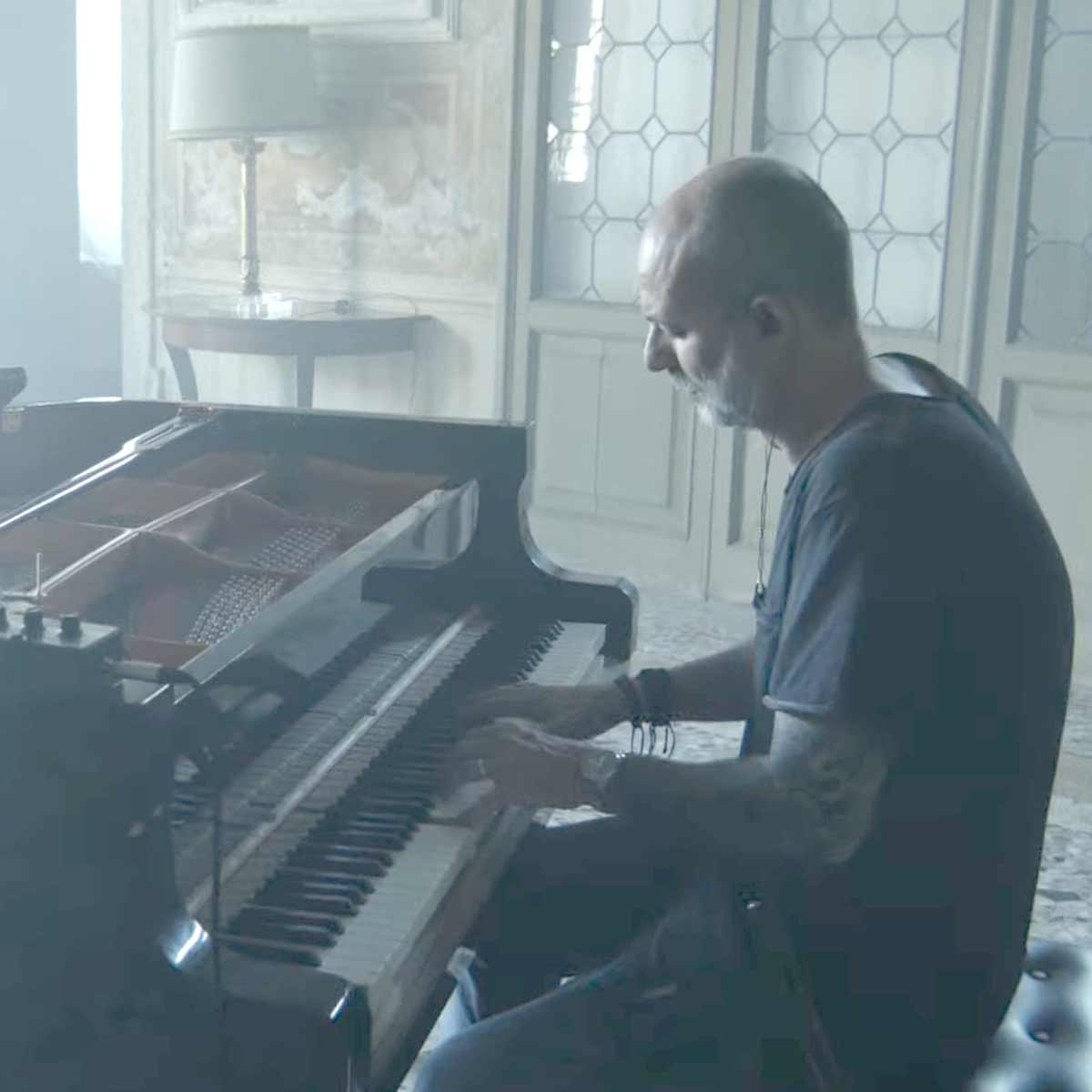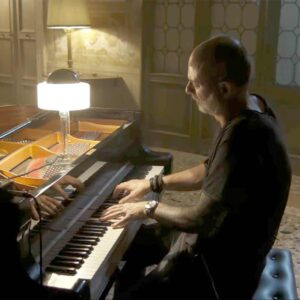# Fabrizio Paterlini

1 Music Sheets

• ###### Birth Name:

Fabrizio Paterlini

• ###### Born:

22 February 1973

• ###### Genres:

Fabrizio Paterlini was born in Mantua, he began playing the piano at the age of six. He studied five years at the Campiani Academy, the Mantua Academy of Arts, where he graduated in Music Theory. The 90s were fruitful for Paterlini in which he performed as a rock, pop and jazz musician in local groups. During this time he began composing music. As the decade and century drew to a close, Paterlini reached an important decision, focusing exclusively on the plane - the instrument which, in his own words, "best expresses his inner world".

Credit: Wikipedia

## Artist's Music Sheets

•e|[tu]|[tu]| w|[tu]|[tu]| 0|[tu]|[tu]| q|[tu]|[tu]| e|[tu]|[tu]| w|[tu]|[tu]| 0|[tu]|[tu]| q|[tu]|[tu] sd [ef]|[tu]|[tu] s [wa]|[tu]|[tu] s [0o]|[tu]|[tu]| [qp]|[tu]|[tu] sd [ef]|[tu]|[tu] s [wa]|[tu]|[tu] s [0o]|[tu]|[tu]| q|[tu]|[tu] sd [ef]|[tu]|[tu] s [ws]a|t|[tu] s [0o]|[tu]|[tu]| [qp]|[tu]|[tu] sd [ef]|[tu]|[tu] s [wa]|[tu]|[tu] s [0o]|[tu]|[tu]| q|[tu]|[tu]| [esl] f [tusl] f [tusl] f [wsl] f [tusl] f [tusl] f [0ak] f [tuak] f [tuak] f [qpj] f [tupj] f [tupj] f [esl] f [tusl] f [tusl] f [wsl] f [tusl] f [tusl] f [0ak] f [tuak] f [tuak] f [qpj] f [tupj] f [tupj] f [efx] j [tufx] j [tufx] j [wfx] h [tufx] h [tufx] h [0dz] h [tudz] h [tudz] h [qsl] g [tusl] g [tusl] g [esl] f [tusl] f [tusl] f [wsl] f [tusl] f [tusl] f [0ak] f [tuak] f [tuak] f [qtup]fj||lz [ex]|[tu]|[tu] l [wl]k|[tu]|[tuk] l [0h]|[tu]|[tu]| [qj]|[tu]|[tu] lz [ex]|[tu]|[tu] l [wk]lk [tu]|[tu] l [0h]|[tu]|[tu]| q|[tu]|[tu] lz [ex]|[tu]|[tu] l [wk]|[tu]|[tu] l [0h]|[tu]|[tu]| [qj]|[tu]|[tu] lz [ex]|[tu]|[tu] l [wk]lk [tu]|[tu] l [0h]|[tu]|[tu]| q|[tu]|[tu]| [elm] x [tulm] x [tulm] x [wlm] x [tulm] x [tulm] x [0kn] x [tukn] x [tukn] x [qjb] x [tujb] x [tujb] x [elm] x [tulm] x [tulm] x [wlm] x [tulm] x [tulm] x [0kn] x [tukn] x [tukn] x [qjb] x [tujb] x [tujb] x [6x] b [tux] b [tux] b [8x] v [wux] v [wux] v [0z] v [toz] v [toz] v [qlm] c [tplm] c [tplm] c [elm] x [tulm] x [tulm] x [wlm] x [tulm] x [tulm] x [0kn] x [tukn] x [tukn] x [qj]lb|| [qtupfj]
Level: 6
Length: 01:49
Intermediate